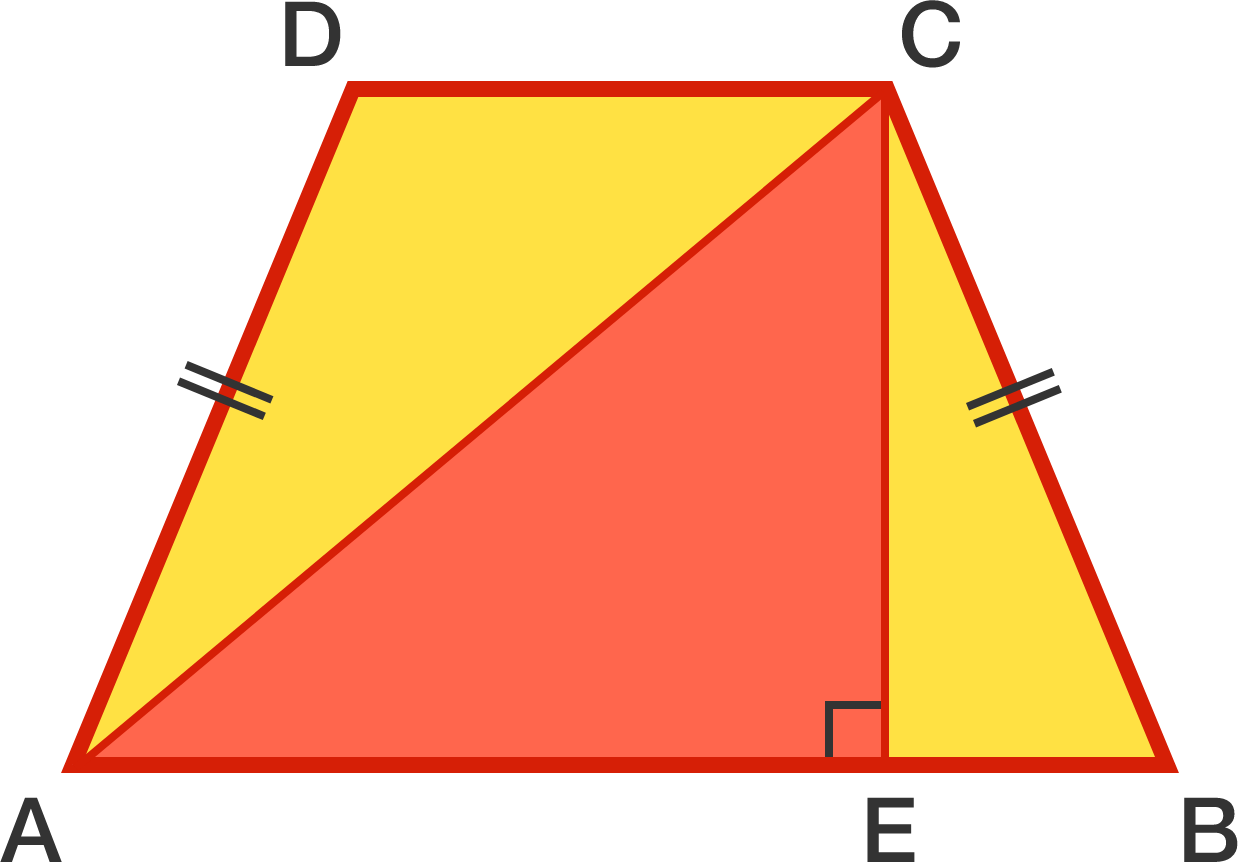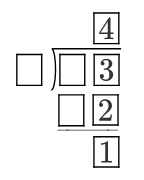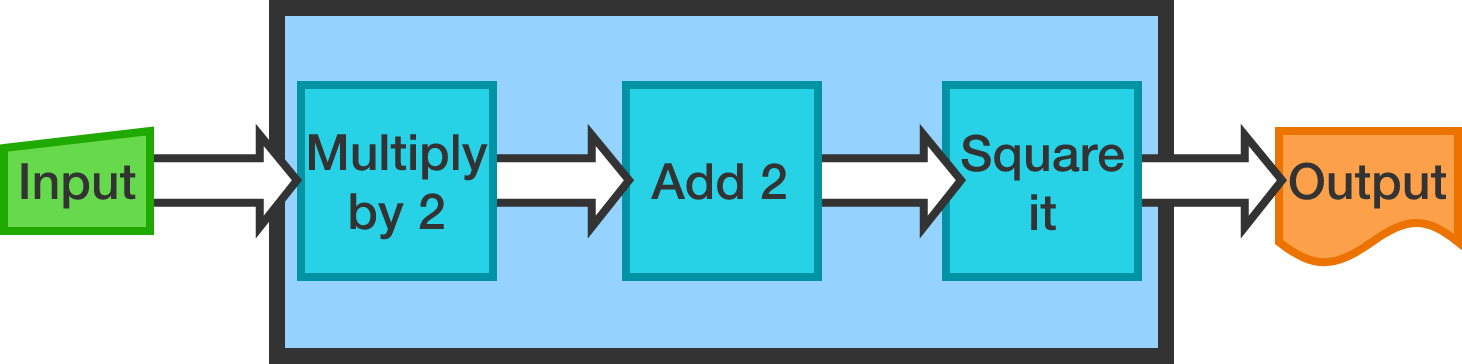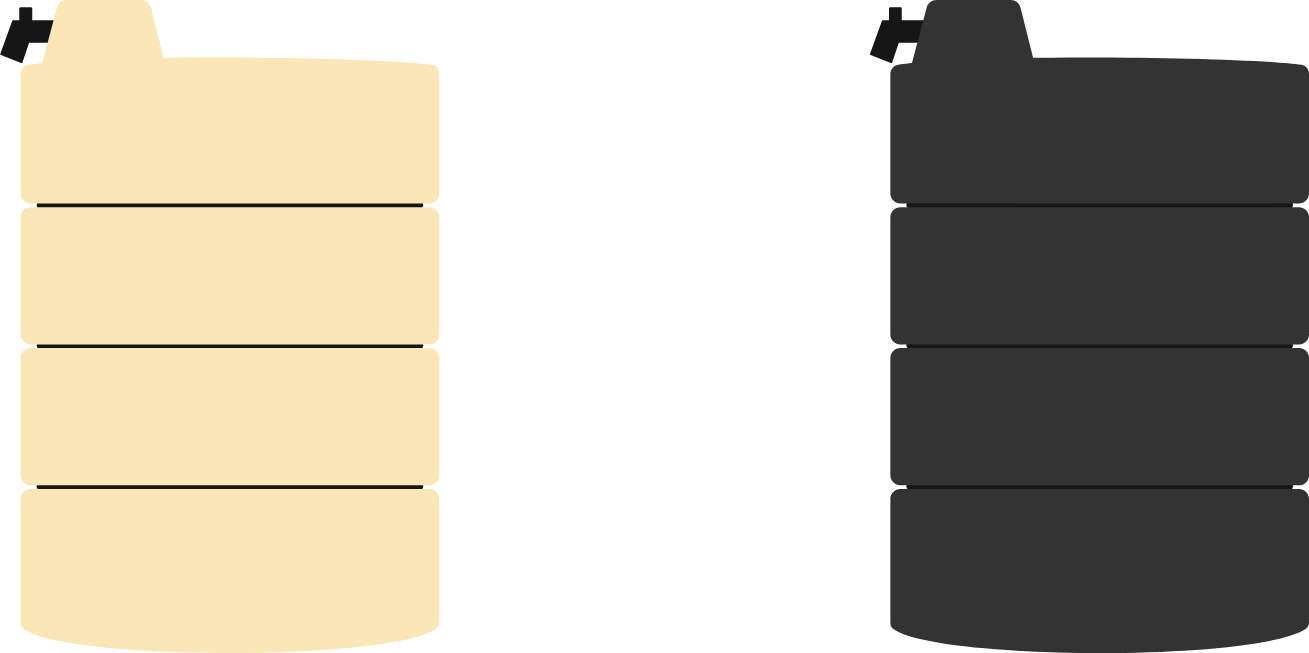# Problems of the Week

Contribute a problem

# 2017-07-10 Basic$ABCD$ is an isosceles trapezoid, and $AEC$ is a right triangle.

Which area is larger, yellow or red?

Below is a long division with some digits hidden. Which of the following digits could represent one of the empty boxes?Alice and Bob have an arithmetic machine. The machine takes in a real number, does some processing and outputs a real number. Specifically, the machine works as follows:Now suppose the following:

• Alice entered a number into the machine and got back 100.
• Bob also entered a number and got back 100.

Does this mean that both Alice and Bob entered the same number?

There is a triplet of positive numbers such that their sum and their product are equal, for example, $(1, 2, 3):$ $1+2+3=1\times 2\times 3.$

True or False?

There are infinitely many triplets of positive numbers (not necessarily integers) such that their sum and their product are equal.

Two identical water tanks with equal volumes of water are placed on a rooftop in a place where it's very hot in the afternoon and very cold at night. One tank is painted dull black on the outside, and the other shiny white.

If we need cooler water during the day, which tank is better suited?×

Problem Loading...

Note Loading...

Set Loading...